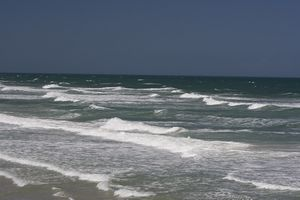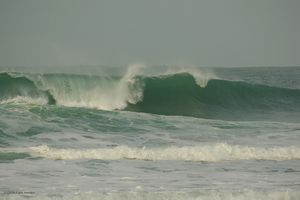# Breaker index

The process of wave breaking plays a crucial role in the morphodynamics of the shoreface and the beach, such as accretion or erosion trends and the development and dynamics of nearshore bars and rip currents. The breaker index concept has been introduced to estimate the location on the shoreface where wave breaking occurs based on empirical formulas, without the need for a detailed simulation of the breaker process.

## A practical wave breaking criterion

According to numerical simulations and flume experiments, wave breaking starts on the shoreface when the horizontal surface water particle velocity at the wave crest comes close (within about 15%) to the wave crest celerity of the tallest wave in the incident wave train. However, it is not easy to derive from this criterion at which location on the shoreface wave breaking will occur. Even if the characteristics of the incident waves and the shoreface profile are well known, one has to face the problem that wave breaking is highly sensitive to fine-scale processes. It cannot be easily modelled, although significant progress has been achieved with numerical techniques such as Computational Fluid Dynamics (CFD) or Smoothed Particle Hydrodynamics (SPH). For this reason, many investigations have been conducted to establish empirical rules for wave breaking from which the location of wave breaking can be easily derived. These empirical rules refer to the so-called breaker index. The breaker index relates the wave height at breaking, $H_b$, to the water depth $h_b$ at the breakpoint; it is defined as

$\gamma_b=H_b/h_b . \qquad (1)$

Important parameters for the wave breaking process are the steepness $H_0/L_0$ of the incident waves ($H_0, L_0$ are the offshore wave height and wavelength) and the average shoreface slope $m$. Empirical rules establish a relationship between the breaker index and these parameters.

## Wave breaking processFig. 1. Schematic of wave evolution towards breaking, for swell waves (left panel) and sea waves (right panel).

The process of wave breaking starts on the shoaling zone where incident waves become progressively skewed by interaction with the seabed: the wave crest becomes sharper and higher, while the wave trough widens and flattens (Fig. 1). Incident waves with short wavelengths (locally generated 'sea') are steeper than incident waves with longer wavelengths (remotely generated swell); the former category of waves will break earlier, thus at greater depth $h_b$ than the latter category, implying a larger value of $\gamma_b$. These short sea waves are also less skewed before breaking; they break when the crest becomes unstable and flows down the front face of the wave, producing spilling breaker bores that surf towards the shoreline (Fig. 2). This breaking mode is also favoured on gently sloping shorefaces when the surf similarity parameter $\xi=m/\sqrt{H_0/L_0}$ has values typically smaller than about 0.4. Incident waves with longer wavelengths will break later, thus at smaller depth $h_b$. While shoaling, they are strongly skewed and break when the crest curls over the front face and falls into the base of the wave, producing a so-called plunging breaker (Fig. 3). This breaking mode is also favoured on steep sloping shorefaces when the surf similarity parameter has values typically larger than 0.4.Fig. 2. Spilling waves. Photo credit Andrew Dawley Flickr Creative Commons.Fig. 3. Plunging wave. Photo credit Kernowfile Flickr Creative Commons.

## Wave breaking formulas

Analytical formulas for the breaker index have been derived from laboratory experiments, field observations and numerical simulation models. Reviews of the various methods and formulas have been given by Camenen and Larson (2007) and Robertson et al. (2013). Most formulas involve the steepness $H_0/L_0$ of the incident wave and the average seabed slope $m$ and reproduce the qualitative tendencies described above, i.e. $\gamma_b$ is an increasing function of the wave steepness and the seabed slope.Fig. 4. The area covered by experimental data for the breaker index as a function of the surf similarity parameter. The density of the experimental data is represented by varying degrees of redness. The black line is the Battjes formula Eq. 4. Figure adapted from Ostendorf and Madsen (1979).

A theoretical limit for $\gamma_b$ was established by Miche (1944), who analysed the wave motion over a horizontal seabed to determine the maximum crest steepness of the incident wave before collapse (occurring when the surface fluid velocity equals the wave crest celerity). The result is

$\gamma_b \lt \Large\frac{0.88}{k_b \, h_b}\normalsize \tanh(k_b\, h_b) , \quad k_b=2 \pi / L_b , \qquad (2)$

where $L_b$ is the wavelength at the breakpoint. This relation was empirically refined by Ostendorf and Madsen (1979) who proposed the formula

$\gamma_b = \Large\frac{0.88}{k_b h_b}\normalsize \tanh(p \, k_b \, h_b) , \quad p = 0.8 + 5 \; min(m, 0.1) . \qquad (3)$

Experiments by Battjes (1974) suggested that the dependence of $\gamma_b$ on wave steepness and bed slope could be represented by the surf similarity parameter $\xi$ at the breakpoint,

$\gamma_b = 1.06 + 0.14 \ln \xi_b . \qquad (4)$

Another frequently used formula was established by Goda (2010),

$\gamma_b = 0.17 \large\frac{L_0}{h_b}\normalsize \Big[1 - \exp[-\large\frac{3 \pi h_b}{2L_0}\normalsize (1+11m^{4/3})] \Big] . \qquad (5)$

The formulas (3) and (5) both require an iterative solution because of the dependence on $h_b$.

The results of experiments designed to determine the breaker index yield widely scattered results, even for experiments with similar bed slope and incident waves. Fig. 4 gives an impression of the range covered by experimental data when the breaker index is plotted as a function of the surf similarity parameter. Although the scatter can be partly explained by the dependence of the breaker index on other parameters besides $\xi$, it also suggests that the breaking process should be considered a stochastic process that is very sensitive to small factors that are not accounted for in the gross characteristics of the experimental setup.

## Irregular waves

The formulas (2-5) were derived for regular monochromatic waves. However, incident wave trains in nature usually have an irregular, random character. An irregular wave field can be characterized by a wave spectrum with significant wave height $H_s$ (see Statistical description of wave parameters). Since individual waves in a wave train have different breakpoints in the surf zone, the breaker index concept cannot be applied as such for $H_s$. This problem can be addressed in two ways. The first way is to consider the breaking of individual waves in an incident wave train, represented by a wave-by-wave breaker index. The second way is to consider the location in the surf zone where $H_s$ starts to decay (the intersection of the shoaling zone where $H_s$ increases and the surf zone where $H_s$ decreases); this location is called the incipient breakpoint, indicated by the subscript $ib$. The wave-by-wave breaker index is similar the breaker index for regular waves. The incipient breaker index $\gamma_{ib}$ is significantly smaller than the breaker index for regular waves, but the dependence on wave steepness and bed slope is similar. The empirical formulas for regular waves can be applied for irregular waves if the value of $\gamma_b$ is reduced by about 30% .

## Wave decay in the surf zone

After initial breaking the wave propagates shoreward, often as a bore, while decreasing in height. The decay of the wave height $H(x)$ can be derived from the equation:

$gradient \; of \; wave \; energy \; flux = wave \; energy \; dissipation, \qquad (6)$

in which for both terms explicit expressions as function $H(x)$ have to be substituted. The wave energy dissipation depends on several factors, including the proportion of broken waves in the surf zone. If all waves are broken, the surf zone is said to be saturated, but for an irregular (random) wave field this is generally not the case. Explicit expressions for the terms in Eq. (6) have been proposed by Battjes and Janssen (1978) and Baldock et al. (1998), but they rely on many assumptions (see the Appendix).

Many laboratory and field investigations have therefore been conducted to establish empirical relationships for the dependence of wave height on water depth in the surf zone,

$H(x)/h(x) = \gamma . \qquad (7)$.

The simplest relationship is a constant ratio of wave height and water depth in the surf zone,

$\gamma (x) = \gamma_b . \qquad (8)$

Flume experiments suggest that this relationship is satisfied to some extent for rather steep shoreface profiles ($m \approx 1/30$), but not for more gently sloping shorefaces. For more gently sloping profiles the ratio $H/h$ displays a notable increase at small water depths. In shallow water, regeneration of waves can take place, which propagate shoreward with less energy loss than wave bores. For irregular waves, the increase of $H_s/h$ is also due to a large percentage of shoaling waves (still increasing in height) after incipient breaking, that gradually decreases as the wave train travels shoreward.

The use of Eq. (7) can be justified if the surf zone depth decreases monotonically towards the shoreline, with an approximately constant slope $m$. In other situations there is no reason to assume that the wave height is related to the local depth. For example, for a wave propagating across a surf zone with nearshore sandbars, the wave height at the trough between sandbars depends on the crest height of the previous sandbar.

## Appendix

Wave energy dissipation in the surf zone can be described by Eq. (6),

$\Large\frac{dP}{dx}\normalsize + D =0 , \qquad (A1)$

where the $x$-axis points towards the local shoreline. In linear wave theory the wave energy flux $P$ can be approximated by

$P= ¼ \rho g H_s^2 c_g \cos \overline \theta , \qquad (A2)$

where $g$ is the gravitational acceleration, $\rho$ the seawater density, $\overline \theta$ the mean incident wave direction and $c_g$ the wave group velocity, which in shallow water is about equal to the wave propagation speed $c \approx \sqrt{gh}$.

An expression for the wave energy dissipation $D$ was derived by Battjes and Janssen (1978) based on analogy with the power dissipated by a bore

$D \approx ¼ Q_b \rho g \Large\frac{H^3}{Th}\normalsize , \qquad (A3)$

where $T$ is the peak wave period, $H$ the wave height and $Q_b$ the fraction of broken waves. Baldock et al. (1998), by assuming a Rayleigh distribution of the incident waves (see Statistical description of wave parameters), derived for $D$ the approximate expression

$D = \Large\frac{\rho g}{4 T}\normalsize \; (H_b^2 + ½ H_s^2 ) \; \exp[-2(H_b/H_s)^2] \, . \qquad (A4)$

An expression of the breaker wave height $H_b$ was derived by Battjes and Janssen (1978) based on Miche's criterion,

$H_b=\Large\frac{0.88}{k}\normalsize \tanh(\large\frac{\gamma}{0.88}\normalsize kh) , \qquad (A5)$

where $k=2 \pi / (cT)$ is the wave number and $\gamma$ is a breaker index ($H_b \approx \gamma h$ in shallow water). By solving Eq. (A1) and comparing the result with data from field surveys on beaches with nearshore sandbars, Ruessink et al. (2003) found for the breaker index $\gamma$ defined by equation (A5) the empirical relationship

$\gamma \approx 0.29+0.76 \, kh . \qquad (A6)$

Zhang et al. (2021) and Myrhaug et al. (2022) provided evidence that the dependence of the breaker index $\gamma$ on $kh$ is in fact a function of the offshore wave steepness.

## Related articles

Shoreface profile
Shallow-water wave theory
Wave transformation
Surf similarity parameter
Waves
Wave set-up
Littoral drift and shoreline modelling